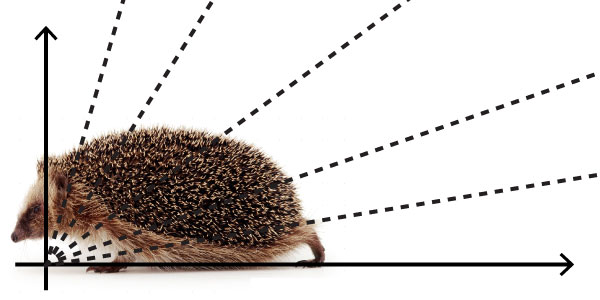# Dividing A Set Of Lines

Probability Level 4Find the smallest positive integer $N$ that has the following property.

Suppose we have a set of $2014$ lines in 3 dimensions that pass through the origin, and no plane contains more than three lines. Then we can divide the lines into $N$ groups, so that every line is perpendicular to at most one line in its group.

Details and assumptions

The statement has to be true for any set of $2014$ lines, and not just a particular set that you chose.

×

Problem Loading...

Note Loading...

Set Loading...